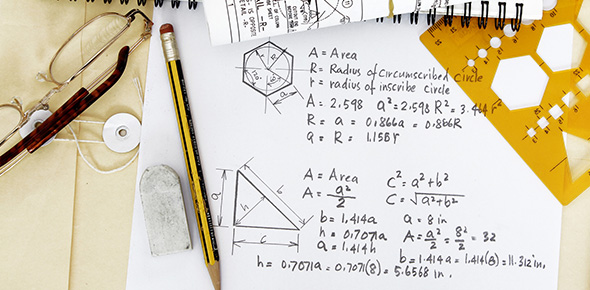# Surface Areas And Volumes

15 Questions | Total Attempts: 1232SettingsSurface areas and volumes of cones, cubes and cylinders are crucial topics in mathematics. Refresh your memory by taking the quiz below to test your knowledge on this interesting topic to see how much you can remember. Enjoy.

• 1.
Volume of the cone is:
• A.

πr2h

• B.

1/3 πr2h

• C.

2/3 πr3

• D.

4/3 πr3

• 2.
What would be the side of a cube of same volume as that of cuboid of 225 m3?
• A.

15 m

• B.

15.5 m

• C.

16.5 m

• D.

14.5 m

• 3.
The total surface area of a solid hemisphere of radius r is:
• A.

πr2

• B.

2πr2

• C.

3πr2

• D.

4πr2

• 4.
The volume and the curved surface area of a sphere are numerically equal, then the radius of sphere is:
• A.

0 units

• B.

1 unit

• C.

2 units

• D.

3 units

• 5.
A cylinder, a cone and a hemisphere are of the same base and of the same height. The ratio of their volumes is:
• A.

1 : 2 : 3

• B.

2 : 1 : 3

• C.

3 : 1 : 2

• D.

3 : 2 : 1

• 6.
Small spheres, each of radius 2cm, are made by melting a solid iron ball of radius 6cm, then the total number of small spheres is:
• A.

6

• B.

9

• C.

27

• D.

81

• 7.
A solid sphere of radius r cm is melted and recast into the shape of solid cone of height r. The radius of the base of the cone is:
• A.

R

• B.

2r

• C.

3r

• D.

4r

• 8.
Three solid spheres of diameters 6 cm. 8 cm and 10 cm are melted to form a single solid sphere. The diameter of the new sphere is:
• A.

3 cm

• B.

4.5 cm

• C.

6 cm

• D.

12 cm

• 9.
The radii of the ends of a frustum of a cone 40 cm high are 38 cm and 8 cm. The slant height of the frustum of cone is:
• A.

26.46 cm

• B.

38.93 cm

• C.

50 cm

• D.

60.96 cm

• 10.
The length of the diagonal of a cube inscribed in a sphere of radius 10 cm is:
• A.

5 cm

• B.

10 cm

• C.

15 cm

• D.

20 cm

• 11.
If a cone is cut into two parts by a horizontal plane passing through the mid-point of the axis, then the ratio of the volumes of the small cone to that of the original cone is
• A.

1 : 2

• B.

1 : 4

• C.

1 : 8

• D.

1 : 16

• 12.
The length of the longest rod that can be placed in a cuboidal room of dimensions 20 cm, 12 cm and 9 cm:
• A.

25 cm

• B.

28 cm

• C.

32 cm

• D.

35 cm

• 13.
A cubical block of side 8 cm is surmounted by a hemisphere. The greatest diameter of the hemisphere is:
• A.

4 cm

• B.

8 cm

• C.

12 cm

• D.

16 cm

• 14.
The number of silver coins, 2 cm in diameter and of thickness 2 mm, is required to melt and form a cuboid of dimension 5 cm X 10 cm X 3 cm is:
• A.

650 coins

• B.

700 coins

• C.

750 coins

• D.

800 coins

• 15.
If the volume of the cube is 729 cm2, then its edge is:
• A.

6 cm

• B.

7 cm

• C.

8 cm

• D.

9 cm

Related Topics